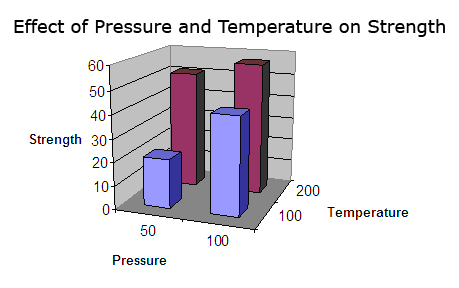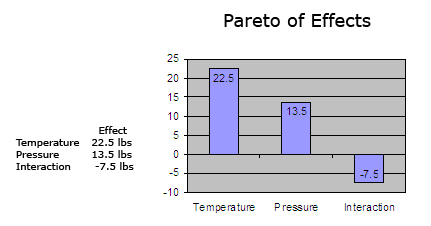Please note: We are currently experiencing high call volumes as we onboard our valued customers to our new system. We appreciate your patience and understanding.
| Cart Total:

# What Is Design of Experiments (DOE)?

Quality Glossary Definition: Design of experiments

Design of experiments (DOE) is defined as a branch of applied statistics that deals with planning, conducting, analyzing, and interpreting controlled tests to evaluate the factors that control the value of a parameter or group of parameters. DOE is a powerful data collection and analysis tool that can be used in a variety of experimental situations.

It allows for multiple input factors to be manipulated, determining their effect on a desired output (response). By manipulating multiple inputs at the same time, DOE can identify important interactions that may be missed when experimenting with one factor at a time. All possible combinations can be investigated (full factorial) or only a portion of the possible combinations (fractional factorial).

A strategically planned and executed experiment may provide a great deal of information about the effect on a response variable due to one or more factors. Many experiments involve holding certain factors constant and altering the levels of another variable. This "one factor at a time" (OFAT) approach to process knowledge is, however, inefficient when compared with changing factor levels simultaneously.

Many of the current statistical approaches to designed experiments originate from the work of R. A. Fisher in the early part of the 20th century. Fisher demonstrated how taking the time to seriously consider the design and execution of an experiment before trying it helped avoid frequently encountered problems in analysis. Key concepts in creating a designed experiment include blocking, randomization, and replication.

• Blocking: When randomizing a factor is impossible or too costly, blocking lets you restrict randomization by carrying out all of the trials with one setting of the factor and then all the trials with the other setting.
• Randomization: Refers to the order in which the trials of an experiment are performed. A randomized sequence helps eliminate effects of unknown or uncontrolled variables.
• Replication: Repetition of a complete experimental treatment, including the setup.

A well-performed experiment may provide answers to questions such as:

• What are the key factors in a process?
• At what settings would the process deliver acceptable performance?
• What are the key, main, and interaction effects in the process?
• What settings would bring about less variation in the output?

A repetitive approach to gaining knowledge is encouraged, typically involving these consecutive steps:

1. A screening design that narrows the field of variables under assessment.
2. A "full factorial" design that studies the response of every combination of factors and factor levels, and an attempt to zone in on a region of values where the process is close to optimization.
3. A response surface designed to model the response.

## When to Use DOE

Use DOE when more than one input factor is suspected of influencing an output. For example, it may be desirable to understand the effect of temperature and pressure on the strength of a glue bond.

DOE can also be used to confirm suspected input/output relationships and to develop a predictive equation suitable for performing what-if analysis.

## Design of Experiments Template and Example

Setting up a DOE starts with process map. ASQ has created a design of experiments template (Excel) available for free download and use. Begin your DOE with three steps:

1. Acquire a full understanding of the inputs and outputs being investigated. A process flowchart or process map can be helpful. Consult with subject matter experts as necessary.
2. Determine the appropriate measure for the output. A variable measure is preferable. Attribute measures (pass/fail) should be avoided. Ensure the measurement system is stable and repeatable.
3. Create a design matrix for the factors being investigated. The design matrix will show all possible combinations of high and low levels for each input factor. These high and low levels can be coded as +1 and -1. For example, a 2 factor experiment will require 4 experimental runs:

 Input A Level Input B Level Experiment #1 -1 -1 Experiment #2 -1 +1 Experiment #3 +1 -1 Experiment #4 +1 +1

Note: The required number of experimental runs can be calculated using the formula 2n, where n is the number of factors.

For each input, determine the extreme (but realistic) high and low levels you wish to investigate. In some cases the extreme levels may be beyond what is currently in use. The extreme levels selected should be realistic, not absurd. For example:

 -1 Level +1 Level Temperature 100 degrees 200 degrees Pressure 50 psi 100 psi
Enter the factors and levels for the experiment into the design matrix. Perform each experiment and record the results. For example:

 Temperature Pressure Strength Experiment #1 100 degrees 50 psi 21 lbs Experiment #2 100 degrees 100 psi 42 lbs Experiment #3 200 degrees 50 psi 51 lbs Experiment #4 200 degrees 100 psi 57 lbs
Calculate the effect of a factor by averaging the data collected at the low level and subtracting it from the average of the data collected at the high level. For example:

Effect of temperature on strength:
(51 + 57)/2 - (21 + 42)/2 = 22.5 lbs

Effect of pressure on strength:
(42 + 57)/2 - (21 + 51)/2 = 13.5 lbs

The interaction between two factors can be calculated in the same fashion. First, the design matrix must be amended to show the high and low levels of the interaction. The levels are calculated by multiplying the coded levels for the input factors acting in the interaction. For example:

 Input A Level Input B Level Interaction Experiment #1 -1 -1 +1 Experiment #2 -1 +1 -1 Experiment #3 +1 -1 -1 Experiment #4 +1 +1 +1
Calculate the effect of the interaction as before. Effect of the interaction on strength: (21 + 57)/2 - (42 + 51)/2 = -7.5 lbs

The experimental data can be plotted in a 3D bar chart.

Design of Experiments: 3D Bar ChartThe effect of each factor can be plotted in a Pareto chart.

Design of Experiments: Pareto ChartThe negative effect of the interaction is most easily seen when the pressure is set to 50 psi and Temperature is set to 100 degrees. Keeping the temperature at 200 degrees will avoid the negative effect of the interaction and help ensure a strong glue bond.

## Conduct and Analyze Your Own DOE

Conduct and analyze up to three factors and their interactions by downloading the design of experiments template (Excel).

## Design of Experiments Summary

More complex studies can be performed with DOE. The above 2-factor example is used for illustrative purposes. A thorough discussion of DOE can be found in Juran’s Quality Handbook.

Contributed by Keith M. Bower.